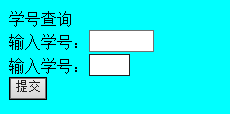<FORM action="" Method="post">
學號查詢
<BR>輸入學號：<Input type=text name="sno" size="5" align="left" maxlength="10">
<BR> 輸入學號：<Input type=text name="sno" size="3" align="left" maxlength="10">

<BR><Input type=submit value="提交">
</FORM>